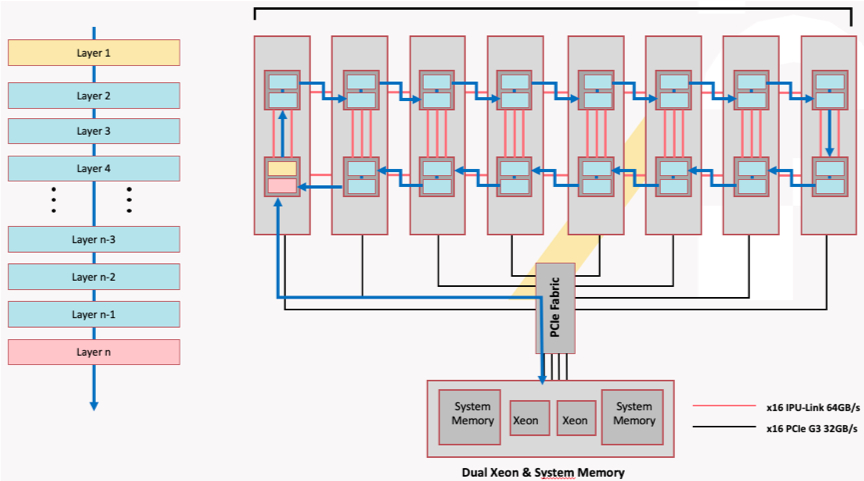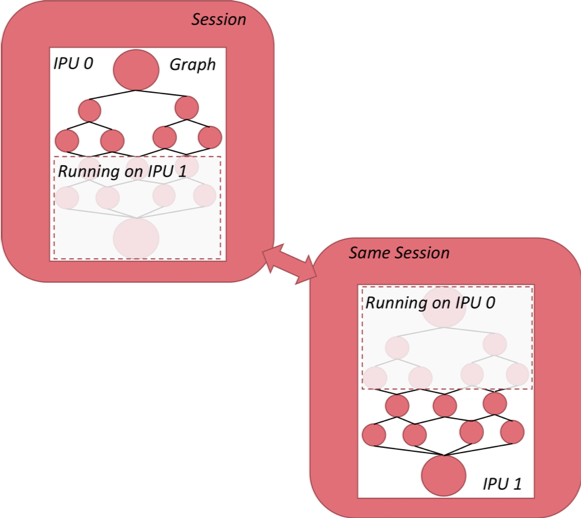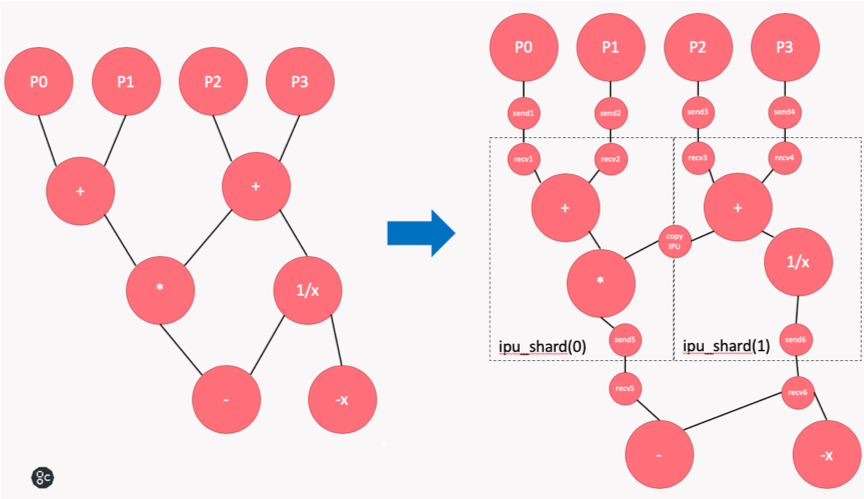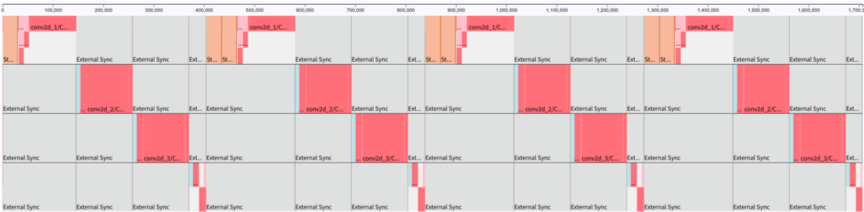# 2. Sharding¶

Sharding means partitioning a neural network, represented as a computational graph, across multiple IPUs, each of which computes a certain part of this graph.

For example, we plan to train a model on an IPU-POD16 DA that has four IPU-M2000s and 16 IPUs. As shown in Fig. 2.1, the model we are training has 32 layers, which cannot fit in one IPU. Logically, we shard the model into 16 subgraphs across the IPUs, where each IPU computes two subgraphs. The direction of the arrows indicates the computation process of the entire model. IPUs communicate with each other through IPU-Links, with bidirectional bandwidth of 64 GB/s.Fig. 2.1 Model sharding over 16 IPUs

Fig. 2.2 shows how we shard a neural network that is implemented in TensorFlow. Even though this model is evenly partitioned across two IPUs and each subgraph can only be visible to its assigned IPU, these two subgraphs are encapsulated within the same session instance and therefore can be trained in a distributed manner.Fig. 2.2 Graph sharding with TensorFlow

Shown on the left in Fig. 2.3 is the computational graph we would like to execute. Let’s say we assign a part of the graph (P0, P1, P2, P3) to the CPU, and partition the rest into two shards across two IPUs. The original computational graph (shown on the left) is transformed into the graph on the right. When the variables required for computation in TensorFlow are distributed on different types of TensorFlow devices (such as CPU and IPU), TensorFlow will add Send and Recv nodes to the graph. If we use sharding, copy nodes will be added between pairs of IPU shards to exchange variables. Copy nodes are implemented with IPU-Link technology.Fig. 2.3 Graph transformation with sharding

## 2.1. Graph sharding¶

We provide an API for manual graph sharding, allowing you to arbitrarily designate sharding points of the graph to achieve maximum flexibility.

### 2.1.1. API¶

The manual model sharding API:

```tensorflow.python.ipu.scopes.ipu_shard(index)
```

Model sharding of a group of operations.

Parameters:

• `index`: IPU index indicates which IPU the operation group is partitioned onto.

### 2.1.2. Code example¶

A code example is shown below:

 ``` 1 2 3 4 5 6 7 8 9 10 11 12 13 14 15 16 17 18 19 20 21 22 23 24 25 26 27 28 29 30 31 32 33 34 35 36 37 38 39 40 41 42 43 44 45``` ```import numpy as np import tensorflow.compat.v1 as tf from tensorflow.python import ipu from tensorflow.python.ipu.scopes import ipu_scope tf.disable_v2_behavior() NUM_IPUS = 4 # Configure the IPU system cfg = ipu.utils.create_ipu_config() cfg = ipu.utils.auto_select_ipus(cfg, NUM_IPUS) ipu.utils.configure_ipu_system(cfg) # Create the CPU section of the graph with tf.device("cpu"): pa = tf.placeholder(np.float32, , name="a") pb = tf.placeholder(np.float32, , name="b") pc = tf.placeholder(np.float32, , name="c") # Distribute the computation across four shards def sharded_graph(pa, pb, pc): with ipu.scopes.ipu_shard(0): o1 = pa + pb with ipu.scopes.ipu_shard(1): o2 = pa + pc with ipu.scopes.ipu_shard(2): o3 = pb + pc with ipu.scopes.ipu_shard(3): out = o1 + o2 + o3 return out # Create the IPU section of the graph with ipu_scope("/device:IPU:0"): result = ipu.ipu_compiler.compile(sharded_graph, [pa, pb, pc]) with tf.Session() as sess: # sharded run result = sess.run(result, feed_dict={ pa: [1., 1.], pb: [0., 1.], pc: [1., 5.] }) print(result) ```

`auto_select_ipus` on line 12 selects four independent IPUs for this task. This function browses the current IPUs in the system in order to determine which IPUs are idle and subscribes to four available IPUs. `sharded_graph()` on lines 22-31 defines a simple graph that consists of some simple additions. Most importantly, the entire graph is partitioned into four shards by calling the `ipu.scopes.ipu_shard()` API four times.

## 2.2. Limitations of sharding¶

Sharding simply partitions the model and distributes it on multiple IPUs. Multiple shards execute in series and cannot fully utilise the computing resources of the IPUs. As shown in Fig. 2.4, the model is partitioned into four parts and executed on four IPUs in series. Only one IPU is working at any one time.Fig. 2.4 Sharding profile

Sharding is a valuable method for use cases that need more control with replication, for example random forests, federated averaging and co-distillation. It can also be useful when developing/debugging a model, however for best performance pipelining should be used in most cases.